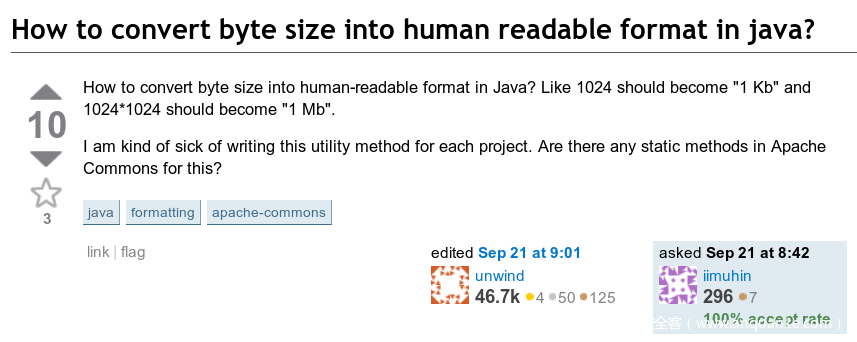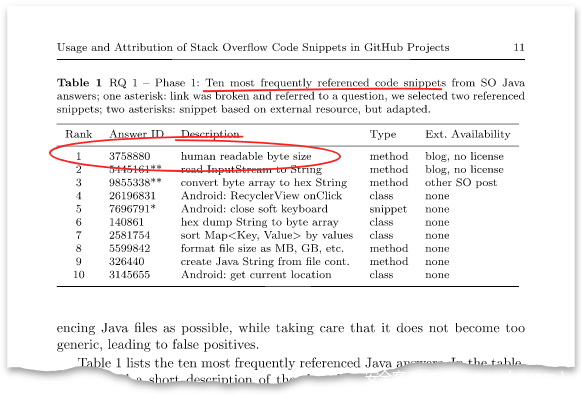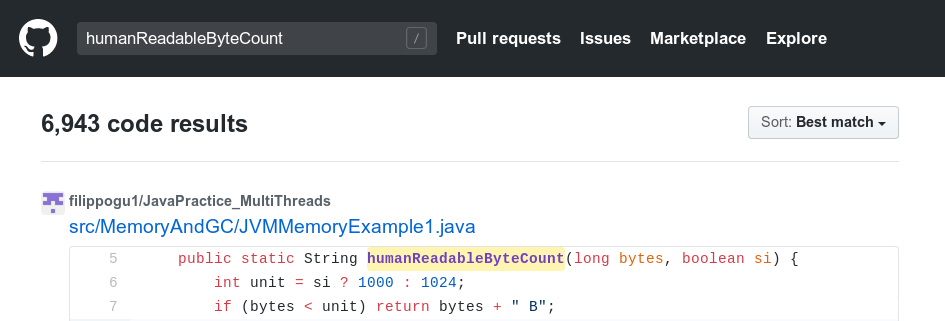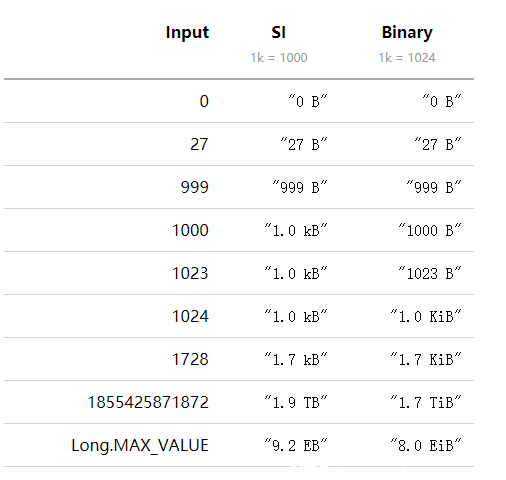Stack Overflow 上有一个 Java 代码片段称霸十年，是 Java 开发人员最爱复制的片段。超过6000个 GitHub Java 项目中复制并内嵌了该代码，远超 Stack Overflow 上的其它Java 片段。该片段的作者本尊Andreas Lundblad 刚刚发布博客文章来修 bug 了……

# 很久以前，久到离谱……``````suffixes   = [ "EB", "PB", "TB", "GB", "MB", "kB", "B" ]
magnitudes = [ 1018, 1015, 1012, 109, 106, 103, 100 ]
i = 0
while (i < magnitudes.length && magnitudes[i] > byteCount)
i++
printf("%.1f %s", byteCount / magnitudes[i], suffixes[i])``````

# 代数神助攻

``````public static String humanReadableByteCount(long bytes, boolean si) {
int unit = si ? 1000 : 1024;
if (bytes < unit) return bytes + " B";
int exp = (int) (Math.log(bytes) / Math.log(unit));
String pre = (si ? "kMGTPE" : "KMGTPE").charAt(exp-1) + (si ? "" : "i");
return String.format("%.1f %sB", bytes / Math.pow(unit, exp), pre);
}``````

# 研究代码署名问题的论文来了

“我们分析了GitHub (GH) 项目中源自 Stack Overflow 上大量 Java 代码片段的使用和署名问题，并给出大规模的实证研究结果。”（提前剧透：多数人在使用时候并未给出署名。）``\$ git grep humanReadableByteCount``

Sebastian 之后就直接找我询问，我答复他说：我发布帖子时还未入职甲骨文，而且我并未负责那个补丁。和甲骨文开玩笑啦。之后不久这个问题就被提交给 OpenJDK，代码也被移除了。

# Bug 来了

``````public static String humanReadableByteCount(long bytes, boolean si) {
int unit = si ? 1000 : 1024;
if (bytes < unit) return bytes + " B";
int exp = (int) (Math.log(bytes) / Math.log(unit));
String pre = (si ? "kMGTPE" : "KMGTPE").charAt(exp-1) + (si ? "" : "i");
return String.format("%.1f %sB", bytes / Math.pow(unit, exp), pre);
}``````

1018兆兆字节之后是1021泽字节。有没有这样一种可能：输入一个很大很大的数时，会导致“Kmgtpe”字符串索引超出范围？并不会。因为最大的 long 值是 263-1≈9.2×1018 ，因此任何 long值都不会超出 EB范围。

# 很多个9

``````if (bytes >= Math.pow(unit, exp) * (unit - 0.05))
exp++;``````

# 更多的9

``````double l1 = Double.MAX_VALUE;
double l2 = l1 - Long.MAX_VALUE;
System.err.println(l1 == l2);  // prints true``````

​ ● String.format 参数中的除法，以及

​ ● 增加 exp 的阈值

# 缩小中间值

``````if (exp > 4) {
bytes /= unit;
exp--;
}``````

# 调整最低有效位

``````long th = (long) (Math.pow(unit, exp) * (unit - 0.05));
if (exp < 6 && bytes >= th - ((th & 0xFFF) == 0xD00 ? 52 : 0))
exp++;``````

# 负值输入

``long absBytes = bytes == Long.MIN_VALUE ? Long.MAX_VALUE : Math.abs(bytes);``

# 最终版本

``````// From: https://programming.guide/the-worlds-most-copied-so-snippet.html
public static strictfp String humanReadableByteCount(long bytes, boolean si) {
int unit = si ? 1000 : 1024;
long absBytes = bytes == Long.MIN_VALUE ? Long.MAX_VALUE : Math.abs(bytes);
if (absBytes < unit) return bytes + " B";
int exp = (int) (Math.log(absBytes) / Math.log(unit));
long th = (long) (Math.pow(unit, exp) * (unit - 0.05));
if (exp < 6 && absBytes >= th - ((th & 0xfff) == 0xd00 ? 52 : 0)) exp++;
String pre = (si ? "kMGTPE" : "KMGTPE").charAt(exp - 1) + (si ? "" : "i");
if (exp > 4) {
bytes /= unit;
exp -= 1;
}
return String.format("%.1f %sB", bytes / Math.pow(unit, exp), pre);
}``````

SI(1 k = 1,000)

``````public static String humanReadableByteCountSI(long bytes) {
String s = bytes < 0 ? "-" : "";
long b = bytes == Long.MIN_VALUE ? Long.MAX_VALUE : Math.abs(bytes);
return b < 1000L ? bytes + " B"
: b < 999_950L ? String.format("%s%.1f kB", s, b / 1e3)
: (b /= 1000) < 999_950L ? String.format("%s%.1f MB", s, b / 1e3)
: (b /= 1000) < 999_950L ? String.format("%s%.1f GB", s, b / 1e3)
: (b /= 1000) < 999_950L ? String.format("%s%.1f TB", s, b / 1e3)
: (b /= 1000) < 999_950L ? String.format("%s%.1f PB", s, b / 1e3)
: String.format("%s%.1f EB", s, b / 1e6);
}``````

Binary (1 K =1,024)

``````public static String humanReadableByteCountBin(long bytes) {
long b = bytes == Long.MIN_VALUE ? Long.MAX_VALUE : Math.abs(bytes);
return b < 1024L ? bytes + " B"
: b < 0xfffccccccccccccL >> 40 ? String.format("%.1f KiB", bytes / 0x1p10)
: b < 0xfffccccccccccccL >> 30 ? String.format("%.1f MiB", bytes / 0x1p20)
: b < 0xfffccccccccccccL >> 20 ? String.format("%.1f GiB", bytes / 0x1p30)
: b < 0xfffccccccccccccL >> 10 ? String.format("%.1f TiB", bytes / 0x1p40)
: b < 0xfffccccccccccccL ? String.format("%.1f PiB", (bytes >> 10) / 0x1p40)
: String.format("%.1f EiB", (bytes >> 20) / 0x1p40);
}``````# 几个启发

​ ● Stack Overflow 上的代码片段可能有 bug，即使获赞数千的答案也不例外。

​ ● 测试所有边缘情况，尤其对于代码是从 Stack Overflow 上复制的情况。

​ ● 浮点运算很难。

​ ● 复制代码时请包括适当的归属说明。不然可能有人会找你哟。